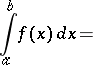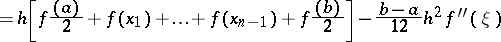# Trapezium formula

(diff) ← Older revision | Latest revision (diff) | Newer revision → (diff)

The special case of the Newton–Cotes quadrature formula in which two nodes are taken: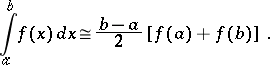(1)

If the integranddiffers strongly from a linear function, then formula (1) is not very exact. In this case the intervalis divided intosubintervals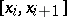,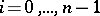, of length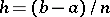, and for the calculation of the integral overone uses the trapezium formula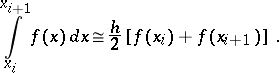Summation of the left- and right-hand sides of this approximate equality with respect tofrom 0 to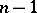leads to the composite trapezium formula: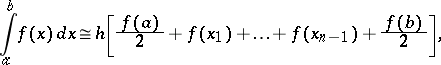(2)

where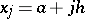,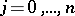. The quadrature formula (2) is also called the trapezium formula (without adding the word composite). The algebraic degree of accuracy of the quadrature formula (2), as well as of (1), is equal to 1. The quadrature formula (2) is exact for the trigonometric functions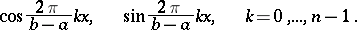In the case when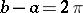, formula (2) is exact for all trigonometric polynomials of order not exceeding; furthermore, its trigonometric degree of accuracy is equal to.

If the integrandis twice-continuously differentiable on, then the error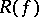of the quadrature formula (2), that is, the difference between the integral and the quadrature sum, is given by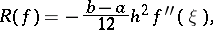whereis a point of.